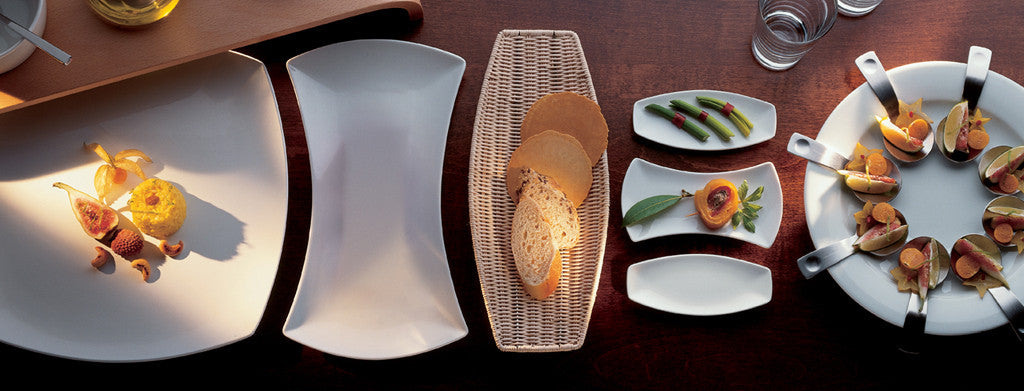Shopping Cart

### Refine

View all

#### Range# Crockery£4.69 each (ex VAT)£2.74 each (ex VAT)£3.25 each (ex VAT)£3.54 each (ex VAT)£4.83 each (ex VAT)£4.66 each (ex VAT)£3.81 each (ex VAT)£1.10 each (ex VAT)£1.22 each (ex VAT)£3.83 each (ex VAT)£3.23 each (ex VAT)£3.26 each (ex VAT)£4.34 each (ex VAT)£4.37 each (ex VAT)£4.67 each (ex VAT)£3.47 each (ex VAT)£3.39 each (ex VAT)£1.95 each (ex VAT)£4.27 each (ex VAT)£2.99 each (ex VAT)£2.07 each (ex VAT)£2.96 each (ex VAT)£3.14 each (ex VAT)£3.92 each (ex VAT)£3.25 each (ex VAT)£2.39 each (ex VAT)£4.02 each (ex VAT)£3.25 each (ex VAT)£2.39 each (ex VAT)£4.63 each (ex VAT)£4.02 each (ex VAT)£4.66 each (ex VAT)£4.66 each (ex VAT)£4.34 each (ex VAT)£4.09 each (ex VAT)£3.12 each (ex VAT)£3.91 each (ex VAT)£2.81 each (ex VAT)£3.21 each (ex VAT)£4.22 each (ex VAT)£2.42 each (ex VAT)£3.26 each (ex VAT)£4.66 each (ex VAT)£3.50 each (ex VAT)£3.88 each (ex VAT)£3.45 each (ex VAT)£4.32 each (ex VAT)£3.57 each (ex VAT)